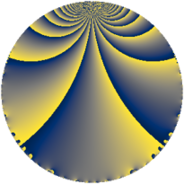Properties

 Label 8280.2.a.baLevel $8280$ Weight $2$ Character orbit 8280.a Self dual yes Analytic conductor $66.116$ Analytic rank $0$ Dimension $2$ CM no Inner twists $1$

Related objects

Newspace parameters

 Level: $$N$$ $$=$$ $$8280 = 2^{3} \cdot 3^{2} \cdot 5 \cdot 23$$ Weight: $$k$$ $$=$$ $$2$$ Character orbit: $$[\chi]$$ $$=$$ 8280.a (trivial)

Newform invariants

 Self dual: yes Analytic conductor: $$66.1161328736$$ Analytic rank: $$0$$ Dimension: $$2$$ Coefficient field: $$\Q(\sqrt{6})$$ Defining polynomial: $$x^{2} - 6$$ Coefficient ring: $$\Z[a_1, \ldots, a_{7}]$$ Coefficient ring index: $$1$$ Twist minimal: yes Fricke sign: $$-1$$ Sato-Tate group: $\mathrm{SU}(2)$

$q$-expansion

Coefficients of the $$q$$-expansion are expressed in terms of $$\beta = \sqrt{6}$$. We also show the integral $$q$$-expansion of the trace form.

 $$f(q)$$ $$=$$ $$q - q^{5} + ( 1 + \beta ) q^{7} +O(q^{10})$$ $$q - q^{5} + ( 1 + \beta ) q^{7} + \beta q^{11} + ( -2 - \beta ) q^{13} + ( 3 - \beta ) q^{17} + \beta q^{19} + q^{23} + q^{25} + ( 3 + 2 \beta ) q^{29} + ( -3 - 2 \beta ) q^{31} + ( -1 - \beta ) q^{35} + ( 1 + 3 \beta ) q^{37} + ( -3 + 2 \beta ) q^{41} + ( 4 - 2 \beta ) q^{43} + ( 4 - \beta ) q^{47} + 2 \beta q^{49} + ( -5 - \beta ) q^{53} -\beta q^{55} + q^{59} + ( 2 + \beta ) q^{61} + ( 2 + \beta ) q^{65} + ( -7 - \beta ) q^{67} + ( 1 + 2 \beta ) q^{71} + ( -6 + \beta ) q^{73} + ( 6 + \beta ) q^{77} + ( 13 + \beta ) q^{83} + ( -3 + \beta ) q^{85} + ( 4 + 2 \beta ) q^{89} + ( -8 - 3 \beta ) q^{91} -\beta q^{95} + ( -6 + 2 \beta ) q^{97} +O(q^{100})$$ $$\operatorname{Tr}(f)(q)$$ $$=$$ $$2 q - 2 q^{5} + 2 q^{7} + O(q^{10})$$ $$2 q - 2 q^{5} + 2 q^{7} - 4 q^{13} + 6 q^{17} + 2 q^{23} + 2 q^{25} + 6 q^{29} - 6 q^{31} - 2 q^{35} + 2 q^{37} - 6 q^{41} + 8 q^{43} + 8 q^{47} - 10 q^{53} + 2 q^{59} + 4 q^{61} + 4 q^{65} - 14 q^{67} + 2 q^{71} - 12 q^{73} + 12 q^{77} + 26 q^{83} - 6 q^{85} + 8 q^{89} - 16 q^{91} - 12 q^{97} + O(q^{100})$$

Embeddings

For each embedding $$\iota_m$$ of the coefficient field, the values $$\iota_m(a_n)$$ are shown below.

For more information on an embedded modular form you can click on its label.

Label $$\iota_m(\nu)$$ $$a_{2}$$ $$a_{3}$$ $$a_{4}$$ $$a_{5}$$ $$a_{6}$$ $$a_{7}$$ $$a_{8}$$ $$a_{9}$$ $$a_{10}$$
1.1
 −2.44949 2.44949
0 0 0 −1.00000 0 −1.44949 0 0 0
1.2 0 0 0 −1.00000 0 3.44949 0 0 0
 $$n$$: e.g. 2-40 or 990-1000 Significant digits: Format: Complex embeddings Normalized embeddings Satake parameters Satake angles

Atkin-Lehner signs

$$p$$ Sign
$$2$$ $$1$$
$$3$$ $$1$$
$$5$$ $$1$$
$$23$$ $$-1$$

Inner twists

This newform does not admit any (nontrivial) inner twists.

Twists

By twisting character orbit
Char Parity Ord Mult Type Twist Min Dim
1.a even 1 1 trivial 8280.2.a.ba 2
3.b odd 2 1 8280.2.a.bg yes 2

By twisted newform orbit
Twist Min Dim Char Parity Ord Mult Type
8280.2.a.ba 2 1.a even 1 1 trivial
8280.2.a.bg yes 2 3.b odd 2 1

Hecke kernels

This newform subspace can be constructed as the intersection of the kernels of the following linear operators acting on $$S_{2}^{\mathrm{new}}(\Gamma_0(8280))$$:

 $$T_{7}^{2} - 2 T_{7} - 5$$ $$T_{11}^{2} - 6$$ $$T_{13}^{2} + 4 T_{13} - 2$$ $$T_{17}^{2} - 6 T_{17} + 3$$

Hecke characteristic polynomials

$p$ $F_p(T)$
$2$ $$T^{2}$$
$3$ $$T^{2}$$
$5$ $$( 1 + T )^{2}$$
$7$ $$-5 - 2 T + T^{2}$$
$11$ $$-6 + T^{2}$$
$13$ $$-2 + 4 T + T^{2}$$
$17$ $$3 - 6 T + T^{2}$$
$19$ $$-6 + T^{2}$$
$23$ $$( -1 + T )^{2}$$
$29$ $$-15 - 6 T + T^{2}$$
$31$ $$-15 + 6 T + T^{2}$$
$37$ $$-53 - 2 T + T^{2}$$
$41$ $$-15 + 6 T + T^{2}$$
$43$ $$-8 - 8 T + T^{2}$$
$47$ $$10 - 8 T + T^{2}$$
$53$ $$19 + 10 T + T^{2}$$
$59$ $$( -1 + T )^{2}$$
$61$ $$-2 - 4 T + T^{2}$$
$67$ $$43 + 14 T + T^{2}$$
$71$ $$-23 - 2 T + T^{2}$$
$73$ $$30 + 12 T + T^{2}$$
$79$ $$T^{2}$$
$83$ $$163 - 26 T + T^{2}$$
$89$ $$-8 - 8 T + T^{2}$$
$97$ $$12 + 12 T + T^{2}$$